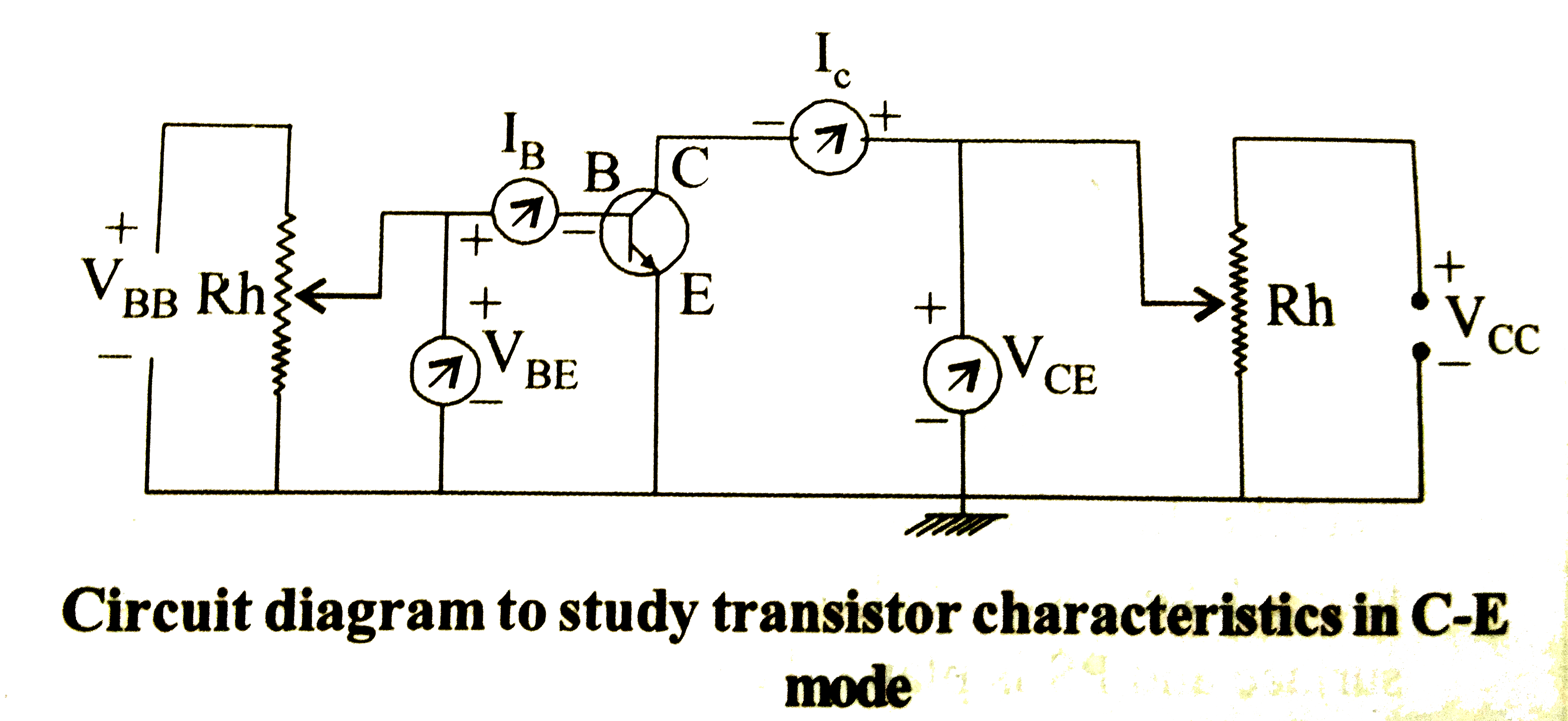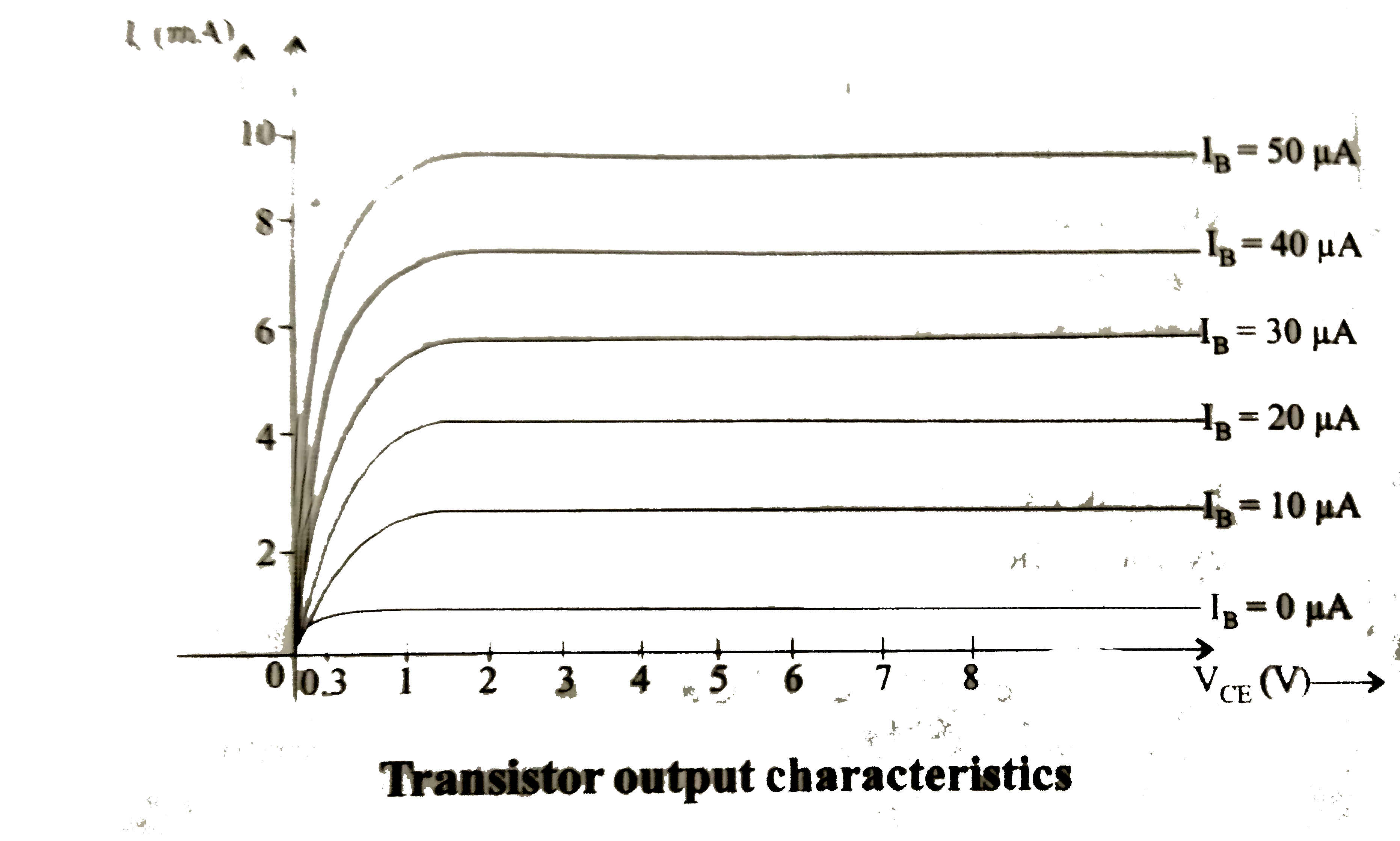# Draw the circuit diagram for studying the characteristics of a transistor in common emitter configuration. Explain briefly and show how input and outp

39 views
in Physics
closed
Draw the circuit diagram for studying the characteristics of a transistor in common emitter configuration. Explain briefly and show how input and output characteristics are drawn.

by (91.7k points)
selectedFor Collector-Emitter mode, it is a graph of collector current (I_(c)) versus collector voltage (V_(CE)), for the fixed value of the base courrent. For output charateristics,I_(B) is kept constant V_(CE) is increased in small steps and I_(C) is recorded at every step.
From output characteristics, it is observed that
(i) When I_(B)=0,I_(C) is nearly zero, hence the trasistor is an cut off region.
(ii) For V_(CE)lt0.3V,I_(C) increases with V_(CE) and can be controlled by V_(CE),
(iii) When V_(CE)gtV_(BE)gt0.7V,C-E jnuction gets reverse baised and I_(C) gets saturated, hence the transistor is in active reagion.
When V_(CE)lt0.2V, both the junctions get forward biased and I_(C) can no longer be controlled by V_(CE), hence the transistor is the saturation region.
The dynamic output resistance of transistor is
r_(0)=((DeltaV_(CE))/(DeltaI_(C)))_(I_(B)" is constant")
In C-E mode, output resistance varies from few KOmega to KOmega.